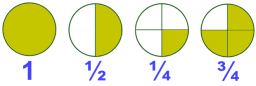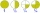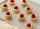What is the sum of 2/3 and 3/10?

x =  0.9667

### Step-by-step explanation:

$x=\frac{2}{3}+\frac{3}{10}=\frac{29}{30}=0.9667$Did you find an error or inaccuracy? Feel free to write us. Thank you!Tips to related online calculators
Need help to calculate sum, simplify or multiply fractions? Try our fraction calculator.

## Related math problems and questions:Add two mixed fractions: 2 4/6 + 1 3/6
• Sum three fractionsWork out the sum of 1/4, 1/5 and 3/10.Why does 1 3/4 + 2 9/10 equal 4.65? How do you solve this?
• Evaluate 11Multiply the quotient of 6 and 2 by 3, then add 1. Add 1 and 6, then divide by 2 and multiply by 3. Divide 6 by the product of 2 and 3, then add 1.Add this two mixed numbers: 1 5/6 + 2 2/11=
• FractionsSort fractions z1 = (6)/(11); z2 = (10)/(21); z3 = (19)/(22) by its size. Result write as three serial numbers 1,2,3.
• Product increasedWhen the product of 2/3 and 6/10 is increased by 2/5, the result is?
• TartletsAfter a special event, a caterer examined the leftovers on the serving table. She saw 10/11 of a tartlet with apples, 2/3 of a tartlet with strawberries, and 10/11 of a tartlet with raspberries. How many leftover tartlets did the caterer have?
• Expression plus minusEvaluate expression: (-1)2 . 12 – 6 : 3 + (-3) . (-2) + 22 – (-3) . 2
• Sum of 20Sum of the two numbers is 15 1/6. If one of the numbers is 2 2/3, find the other number.What is 4 1/2+2/7-213/14?What is the sum of 2/3+3/5?What is the quotient of 3/10 divided by 2/4 as a fraction?Add marks (+, -, *, /, brackets) to fullfill equations 1 3 6 5 = 10 This is for the 4th grade of the primary school - with no negative numbers yetThe product of two numbers are 2/3. If on of them is 1/10, what is the other?The sum of 3 mixed numbers is 20 13/15. two of the numbers are 6 1/3 and 7 5/6. what is the third number?Add this two mixed numbers: 1 5/6 + 2 2/11=